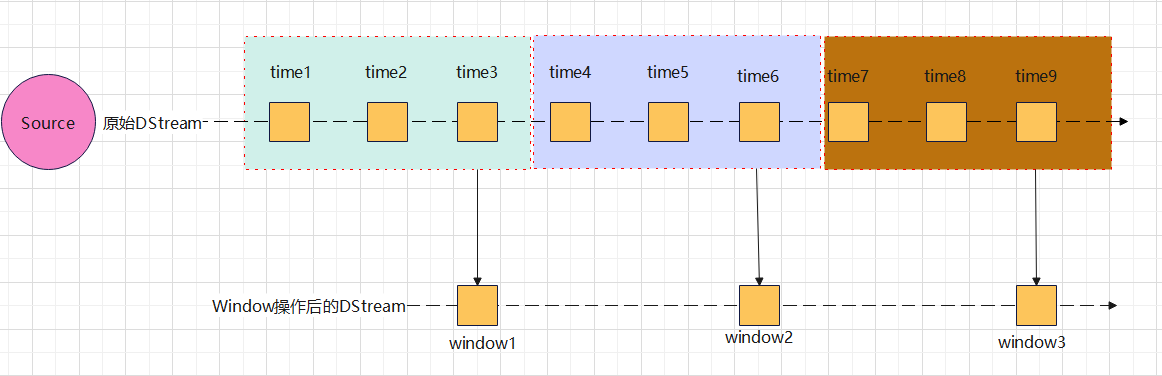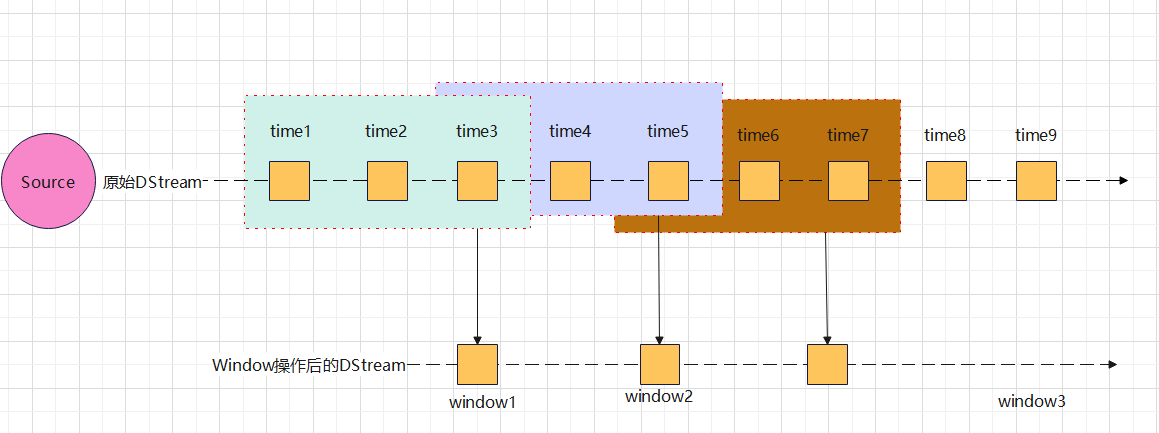# window overview of Spark Dstream

Spark DStream provides Window operations, and we can use Window operators to perform a series of operator operations on the data. Unlike Flink, the window operations provided by Spark DStream are relatively simple. Operations can only be performed based on the processing time of the data. Spark's windows can be divided into two categories: rolling windows and sliding windows.

• scroll windowAs can be seen from the above figure, the rolling window has the following characteristics:

• The size of the window is a fixed size interval
• There is no intersection between windows.
• The step size of each sliding of the window is equal to the size of the window
• sliding window• The size of the window is a fixed size interval
• There is an intersection between windows and windows.
• The step size of each sliding of the window is less than the size of the window

If the sliding step size of the window is larger than the size of the window, some data may be lost.

Tips: The window size of Spark must be an integer multiple of the batch time, and the sliding step size of the window must also be an integer multiple of the batch time, because Spark Streaming is not a real real-time stream processing framework in the strict sense (to process one by one), The smallest unit of data processing is one batch. Therefore, if the size of the window or the sliding step size is not an integer multiple of the batch time, then the batch will be split into multiple parts.

# Window API operation

## window(windowLength,slideInterval)

• Description: Creates a window operation based on a DStream of data.
• windowLength: is the size of the window
• slideInterval: is the sliding step size

windowsLength and slideInterval must be an integer multiple of the batch time of the set data stream

## countByWindow(windowLength, slideInterval)

• Description: Count the number of elements in the sliding window.
• windowLength: is the size of the window
• slideInterval: is the sliding step size

windowsLength and slideInterval must be an integer multiple of the batch time of the set data stream

## reduceByWindow(func, windowLength, slideInterval)

• Description: Create a window, perform a reduce operation on each element in the window, and return a Stream with only a single element.
• func reduce function
• windowLength: is the size of the window
• slideInterval: is the sliding step size

windowsLength and slideInterval must be an integer multiple of the batch time of the set data stream

• Description: Create a window for a DStream of type (K,V), group the windows by Key, perform a reduce operation on each element in the group, and return a DStream of type (K,V).
• func reduce function
• windowLength: is the size of the window
• slideInterval: is the sliding step size

windowsLength and slideInterval must be an integer multiple of the batch time of the set data stream

• Description: Create a window for a DStream of type (K, V), group the elements in the window according to Key, count the number of V for each element in the group, and return a DStream of type (K,Long).
• windowLength: is the size of the window
• slideInterval: is the sliding step size

windowsLength and slideInterval must be an integer multiple of the batch time of the set data stream

Tips: All the above windows operators are "lazy execution" operators.

```package com.hjt.yxh.hw.dstream

import org.apache.spark.streaming.{Seconds, StreamingContext}
import org.apache.spark.{SparkConf, SparkContext}

object WindowTestApp {
def main(args: Array[String]): Unit = {

val sparkConf :SparkConf = new SparkConf()
sparkConf.setMaster("local[*]").setAppName("DStreamTestApp")
val ssc:StreamingContext = new StreamingContext(sparkConf,Seconds(3))

import StreamingContext._

.filter(_.nonEmpty)
.map(data=>{
val arr = data.split(",")
})

//Rolling window, the size of the window is 9 seconds, and the time of the sensor with the highest temperature in each window is counted
val maxDStream:DStream[(String,SensorReading)] = sensorDs.window(Seconds(9)).reduceByKey((first, second) => {
if (first.temperature > second.temperature) {
first
} else {
second
}
})

//output to console
maxDStream.print()

ssc.start()
ssc.awaitTermination()

}
}

```

Tags: Big Data Spark

Posted by zrosen88 on Wed, 07 Sep 2022 08:42:23 +0300